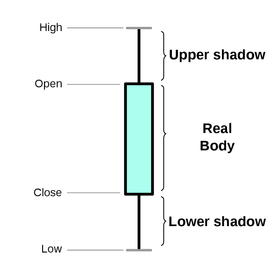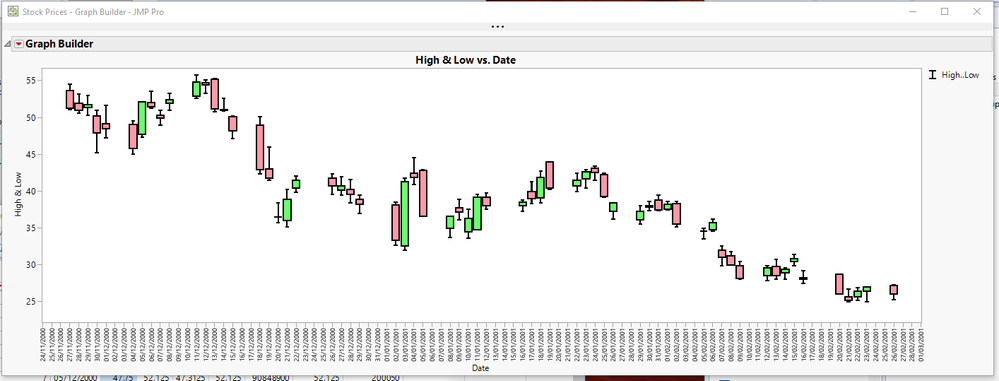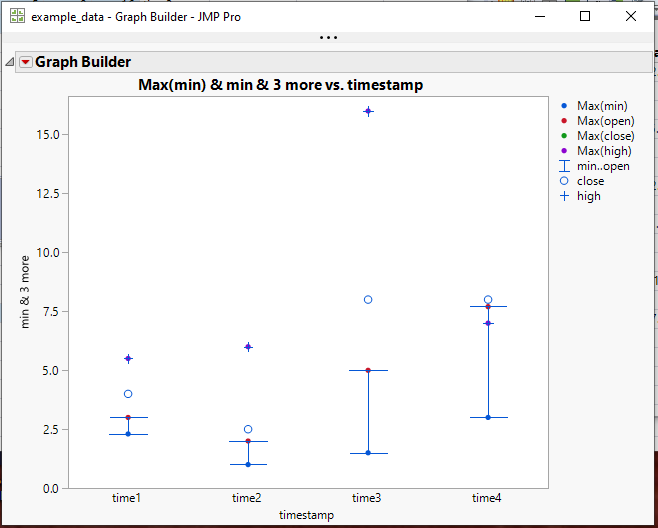Our World Statistics Day conversations have been a great reminder of how much statistics can inform our lives. Do you have an example of how statistics has made a difference in your life? Share your story with the Community!
Choose Language Hide Translation Bar
Highlighted

## Presenting stock data in form of box plot

Hello Everybody,

the data describing stock price movements is created by open/close price and max/min price. Is there any way to create box plot (candlestick chart) in JMP having real body from open and close price and upper and lower shadow having values in max (high) and min (low) prices respectively, like in the picture attached or is there better way to do that? The traditional Box Plot element, however, shows outlier or quantile box plots and provides a compact view of a distribution of values. I attached an example data table in which several timestamps and price values (min, open, close and high) are provided. I would appreciate for hints and suggestions.(source: Wikipedia)

Best regards

1 ACCEPTED SOLUTION

Accepted Solutions
Highlighted

## Re: Presenting stock data in form of box plot

I had a little more time to look into this, and I think the easy solution below might be a workable solution for you.``````Names Default To Here( 1 );
dt = Open( "\$SAMPLE_DATA/Stock Prices.jmp" );

dt:open << label;
dt:close << label;

gb = dt << Graph Builder(
Size( 1289, 456 ),
Show Control Panel( 0 ),
Variables( X( :Date ), Y( :High ), Y( :Low, Position( 1 ) ) ),
Elements( Bar( X, Y( 1 ), Y( 2 ), Legend( 10 ), Bar Style( "Interval" ) ) ),
SendToReport(
Dispatch(
{},
"Date",
ScaleBox,
{ Interval( "Day" ), Inc( 1 ),
Minor Ticks( 0 )}
),
Dispatch(
{},
"400",
ScaleBox,
{Legend Model(
10,
Properties( 0, {Line Width( 2 )}, Item ID( "High..Low", 1 ) )
)}
)
)
);

// Add the boxes to the chart
Report( gb )[framebox( 1 )] << add graphics script(
halfDayincr = In Days( 1 ) / 2 * .8;
days = (Col Max( :Date ) - Col Min( :Date )) / In Days( 1 );
Pen Color( black );
Pen Size( 2 );
For( i = 1, i <= N Rows( dt ), i++,
If( :Open[i] <= :close[i],
Fill Color( light green );
,
Fill Color( light red );
);
Rect( :date[i] - halfDayincr, Max( :open[i], :close[i] ), :date[i] + halfDayincr, Min( :open[i], :close[i] ), 1 );
Rect( :date[i] - halfDayincr, Max( :open[i], :close[i] ), :date[i] + halfDayincr, Min( :open[i], :close[i] ), 0 );
);
);

``````
Jim
6 REPLIES 6
Highlighted

## inkRe: Presenting stock data in form of box plot

I think this is the best that JMP can do without scriptingBut with a little bit of effort, one could easily write some JSL that would create the Candlestick plots.

Jim
Highlighted

## Re: Presenting stock data in form of box plot

Yes, as Jim points out, Graph Builder can do this easily.  As you get more data, though, you might want to consider IR (individual moving range charts) as these will provide insight into consistency/stability of the metrics over time.

Highlighted

## Re: Presenting stock data in form of box plot

I had a little more time to look into this, and I think the easy solution below might be a workable solution for you.``````Names Default To Here( 1 );
dt = Open( "\$SAMPLE_DATA/Stock Prices.jmp" );

dt:open << label;
dt:close << label;

gb = dt << Graph Builder(
Size( 1289, 456 ),
Show Control Panel( 0 ),
Variables( X( :Date ), Y( :High ), Y( :Low, Position( 1 ) ) ),
Elements( Bar( X, Y( 1 ), Y( 2 ), Legend( 10 ), Bar Style( "Interval" ) ) ),
SendToReport(
Dispatch(
{},
"Date",
ScaleBox,
{ Interval( "Day" ), Inc( 1 ),
Minor Ticks( 0 )}
),
Dispatch(
{},
"400",
ScaleBox,
{Legend Model(
10,
Properties( 0, {Line Width( 2 )}, Item ID( "High..Low", 1 ) )
)}
)
)
);

// Add the boxes to the chart
Report( gb )[framebox( 1 )] << add graphics script(
halfDayincr = In Days( 1 ) / 2 * .8;
days = (Col Max( :Date ) - Col Min( :Date )) / In Days( 1 );
Pen Color( black );
Pen Size( 2 );
For( i = 1, i <= N Rows( dt ), i++,
If( :Open[i] <= :close[i],
Fill Color( light green );
,
Fill Color( light red );
);
Rect( :date[i] - halfDayincr, Max( :open[i], :close[i] ), :date[i] + halfDayincr, Min( :open[i], :close[i] ), 1 );
Rect( :date[i] - halfDayincr, Max( :open[i], :close[i] ), :date[i] + halfDayincr, Min( :open[i], :close[i] ), 0 );
);
);

``````
Jim
Highlighted

## Re: Presenting stock data in form of box plot

Thank you very much! I tried to use your code on the data from yahoo finance website, however, the data from there is saved as characters with "." decimal points which did not allow to convert values to correct numeric values. The below snippet converts values in columns and allows to plot them correctly. Thank you and best regards.

``````//open data

//change decimal point from "." to ",", otherwise later conversion to numeric format produces wrong results
nr = nrows(dt);
for (k = 1, k <= nr, k++,
dt:Open[k] = substitute(dt:Open[k], ".", ",");
dt:Close[k] = substitute(dt:Close[k], ".", ",");
dt:High[k] = substitute(dt:High[k], ".", ",");
dt:Low[k] = substitute(dt:Low[k], ".", ",");
);

//change format to numeric
dt:Open << Data Type( numeric ) << Modeling Type( continuous );
dt:Close << Data Type( numeric ) << Modeling Type( continuous );
dt:High << Data Type( numeric ) << Modeling Type( continuous );
dt:Low << Data Type( numeric ) << Modeling Type( continuous );
dt:name("Adj Close") << Data Type( numeric ) << Modeling Type( continuous );``````
Highlighted

## Re: Presenting stock data in form of box plot

What you found with the data returned from the Yahoo Finance data must be Country settings specific. The data that was returned to me in the U.S. did not have to be converted.
Jim
Highlighted

## Re: Presenting stock data in form of box plot

OK, thank you, I will notice that. Best regards

Article Labels